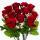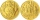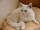Store

Peter paid in store 3 euros more than half the amount that was on arrival to the store. When he leave shop he left 10 euros. How many euros he had upon arrival to the store?

Result

x =  26 Eur

Solution:

(3+x/2) +10= x

x = 26

x = 26

Calculated by our simple equation calculator.

Leave us a comment of this math problem and its solution (i.e. if it is still somewhat unclear...):Be the first to comment!To solve this verbal math problem are needed these knowledge from mathematics:

Do you have a linear equation or system of equations and looking for its solution? Or do you have quadratic equation?

Next similar math problems:

1. 7 rosesPeter buys 7 roses. When he pay for it left him 4 euro. If he bought 5 roses left him 40 euros. How many euros had Peter before buying?
2. CandyPeter had a sachet of candy. He wanted to share with his friends. If he gave them 30 candies, he would have 62 candies. If he gave them 40 candies, he would miss 8 candies. How many friends did Peter have?
3. Far countryIn a country far away, the value of 3 pesos is 12 centavos more than the value of 1 peso. How many centavos is 1 peso worth?
4. Fifth of the numberThe fifth of the number is by 24 less than that number. What is the number?
5. Tickets 3A total of 645 tickets were sold for the school play. They were either adult tickets or student tickets. There were 55 fewer student tickets sold than adult tickets. How many adult tickets were sold?
6. CatOne-fifth of the monthly pocket money contributes Maros for food for his cat, half of the rest postpone for a new smartphone. The remaining € 8 spend. How much pocket money gets Maros a month?
7. Unknown number 11That number increased by three equals three times itself?
8. InfirmaryTwo thirds of children from the infirmary went on a trip seventh went to bathe and 40 children remained in the gym. How many children were treated in the infirmary?
9. Simple equationSolve the following simple equation: 2. (4x + 3) = 2-5. (1-x)
10. One-thirdA one-third of unknown number is equal to five times as great as the difference of the same unknown number and number 28. Determine the unknown number.
11. Simple equation 8Solve the following equation: 36=-(1+7x)-6(-7-x)
12. Apples 2How many apples were originally on the tree, if the first day fell one third, the second day quarter of the rest and on tree remained 45 apples?
13. Simple equation 9Solve the following equation: -8y+5=-9y+9
14. Theorem proveWe want to prove the sentence: If the natural number n is divisible by six, then n is divisible by three. From what assumption we started?
15. Dropped sheetsThree consecutive sheets dropped from the book. The sum of the numbers on the pages of the dropped sheets is 273. What number has the last page of the dropped sheets?
16. EquationSolve the equation: 1/2-2/8 = 1/10; Write the result as a decimal number.
17. Equation?